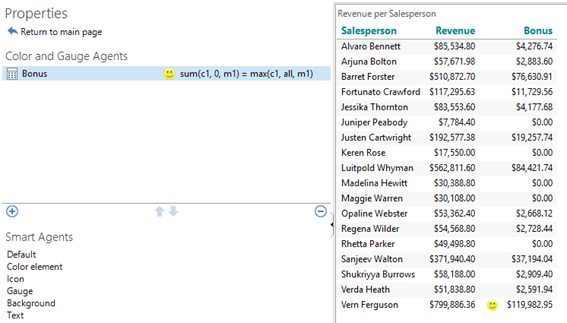# A “nested” If-then-else

Maybe more calculations need to be done depending on different conditions.

This can be solved using a “nested” if-sentence.

• Create a new Bonus analysis with a crosstab showing Revenue per Salesperson.
• Add a dynamic criteria = Previous month

Now we’ve got a basis for calculating the monthly bonus for the Salespersons. The bonus is given according to these rules:

• Revenue 0-50000 = 0 in bonus
• Revenue > 50000 = 5% of Revenue in bonus
• Revenue >= 100000 and < 500000 = 10% of Revenue in bonus
• Revenue >= 500000 = 15% of Revenue in bonus

This can be translated to Targit calculation syntax using one long nested if sentence:

if sum(d1, 0, m1) >= 500000 then sum(d1, 0, m1) * 0.15 else
if sum(d1, 0, m1) >= 100000 then sum(d1, 0, m1) * 0.1 else
if sum(d1, 0, m1) >= 50000 then sum(d1, 0, m1) * 0.05 else 0

Notice: The last else takes care of those who do not meet any of the conditions and as a result has no bonus coming.

if SALES:(sum(d1, 0, m1)) >= 500000 then SALES * 0.15 else
if SALES >= 100000 then SALES * 0.1 else
if SALES >= 50000 then SALES * 0.05 else 0

Make sure that an icon agent highlights the salesperson who recieves the highest bonus.

With June 2019 set as the dynamic date origin the crosstab should look like this: